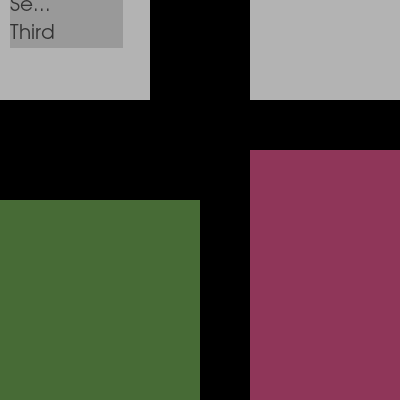# Using Layouts with different UI elements#

This example shows how to place different UI elements in different Layouts. The Layouts used here is GridLayout (with different cell shapes).

First, some imports.

```from fury import ui, window
from fury.layout import GridLayout
```

We create some panels and then we arrange them in a grid fashion

First, we create some panels with different sizes/positions

```panel_1 = ui.Panel2D(size=(200, 200), color=(0.4, 0.6, 0.3), position=(100, 100))

panel_2 = ui.Panel2D(size=(250, 250), color=(0.8, 0.3, 0.5), position=(150, 150))
```

Now we create two listboxes

```listbox_1 = ui.ListBox2D(size=(150, 150), values=['First', 'Second', 'Third'])

listbox_2 = ui.ListBox2D(size=(250, 250), values=['First', 'Second', 'Third'])
```

Now we create two different UI i.e. a slider and a listbox

```slider = ui.LineSlider2D(length=150)
listbox = ui.ListBox2D(size=(150, 150), values=['First', 'Second', 'Third'])
```

Now, we create grids with different shapes

```rect_grid = GridLayout(position_offset=(0, 0, 0))
square_grid = GridLayout(cell_shape='square', position_offset=(0, 300, 0))
diagonal_grid = GridLayout(cell_shape='diagonal', position_offset=(0, 600, 0))
```

Applying the grid to the ui elements

```rect_grid.apply([panel_1, panel_2])
square_grid.apply([listbox_1, listbox_2])
diagonal_grid.apply([slider, listbox])

current_size = (1500, 1500)
show_manager = window.ShowManager(size=current_size, title='FURY UI Layout')

show_manager.scene.add(panel_1, panel_2, listbox_1, listbox_2, slider, listbox)

# To interact with the UI, set interactive = True
interactive = False

if interactive:
show_manager.start()

window.record(show_manager.scene, out_path='ui_layout.png', size=(400, 400))
```Total running time of the script: ( 0 minutes 0.066 seconds)

Gallery generated by Sphinx-Gallery